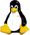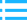﻿ Linux下多線程編程互斥鎖和條件變量的簡單使用_Linux編程Linux教程網Linux教程網 >> Linux編程 >> Linux編程 >> Linux下多線程編程互斥鎖和條件變量的簡單使用

# Linux下多線程編程互斥鎖和條件變量的簡單使用

#include <iostream>
#include <unistd.h>

using namespace std;

void* run(void* para)
{

//sleep(5);//suspend 5 s，在正式的代碼中，一般不要用sleep函數
int* iptr = (int*)((void**)para);
float* fptr = (float*)((void**)para);
char* str = (char*)((void**)para);
cout << *iptr << "    " << *fptr << "    " << str << endl;

return ((void *)0);
}

int main()
{
int err = -1;
int ival = 1;
float fval = 10.0F;
char buf[] = "func";
void* para = { &ival, &fval, buf };

err = pthread_create(&pid, NULL, run, para);
if (err != 0) {
cout << "can't create thread!" << endl;
return -1;
}

//新線程創建之後主線程如何運行----主線程按順序繼續執行下一行程序
cout << "main thread!" << endl;

//新線程結束時如何處理----新線程先停止，然後作為其清理過程的一部分，等待與另一個線程合並或“連接”

cout << "ok!" << endl;

return 0;
}

#include <iostream>

using namespace std;

int counter = 0;

void* run(void* arg)
{

unsigned long i = 0;
counter += 1;
cout << "Job " << counter << " started!" << endl;
for (i = 0; i<(0xFFFFFFFF); i++);
cout << "Job " << counter << " finished!" << endl;

return NULL;
}

int main()
{
int i = 0, err = -1;

if (pthread_mutex_init(&lock, NULL) != 0) {
cout << "mutex init failed" << endl;
return -1;
}

while (i < 2) {
err = pthread_create(&(tid[i]), NULL, &run, NULL);
if (err != 0)
cout << "can't create thread!" << endl;
i++;
}

cout << "ok!" << endl;
return 0;
}

#include <iostream>
#include <unistd.h>

using namespace std;

unsigned count = 0;

void* decrement_count(void* arg)
{
cout << "decrement_count get count_lock" << endl;

while (count == 0) {
cout << "decrement_count count == 0" << endl;

cout << "decrement_count before cond_wait" << endl;
cout << "decrement_count after cond_wait" << endl;
}

count = count + 1;

return NULL;
}

void* increment_count(void* arg)
{
cout << "increment_count get count_lock" << endl;

if (count == 0) {
cout << "increment_count before cond_signal" << endl;
cout << "increment_count after cond_signal" << endl;
}

count = count + 1;

return NULL;
}

int main()
{

sleep(2);

sleep(2);

cout << "ok!" << endl;
}

#include <iostream>
#include <unistd.h>

using namespace std;

int counter = 0;

void* decrement_counter(void* argv);
void* increment_counter(void* argv);

int main()
{
cout << "counter: " << counter << endl;

int ret = -1;

ret = pthread_create(&thd1, NULL, decrement_counter, NULL);
if (ret){
cout << "create thread1 fail" << endl;
return -1;
}

ret = pthread_create(&thd2, NULL, increment_counter, NULL);
if (ret){
cout << "create thread2 fail" << endl;
return -1;
}

int counter = 0;
while (counter != 10) {
cout << "counter(main): " << counter << endl;
sleep(1);
counter++;
}

cout << "ok!" << endl;
}

void* decrement_counter(void* argv)
{
cout << "counter(decrement): " << counter << endl;

while (counter == 0)

cout << "counter--(decrement, before): " << counter << endl;
counter--; //等待signal激活後再執行
cout << "counter--(decrement, after): " << counter << endl;

return NULL;
}

void* increment_counter(void* argv)
{
cout << "counter(increment): " << counter << endl;

if (counter == 0)

cout << "counter++(increment, before): " << counter << endl;
counter++;
cout << "counter++(increment, after): " << counter << endl;

return NULL;
}

#include <iostream>
#include <unistd.h>

using namespace std;

int counter = 0;

void* decrement_increment_counter(void* argv);

int main()
{
cout << "counter: " << counter << endl;

int ret = -1;

ret = pthread_create(&thd, NULL, decrement_increment_counter, NULL);
if (ret){
cout << "create thread1 fail" << endl;
return -1;
}

int counter = 0;
while (counter != 10) {
cout << "counter(main): " << counter << endl;
sleep(1);
counter++;
}

cout << "ok!" << endl;
}

void* decrement_increment_counter(void* argv)
{
cout << "start counter: " << counter << endl;

cout << "counter(decrement): " << counter << endl;
while (counter == 1)

cout << "counter--(decrement, before): " << counter << endl;
counter--; //等待signal激活後再執行
cout << "counter--(decrement, after): " << counter << endl;

cout << "counter(increment): " << counter << endl;
if (counter == 0)

cout << "counter++(increment, before): " << counter << endl;
counter++;
cout << "counter++(increment, after): " << counter << endl;

return NULL;
}